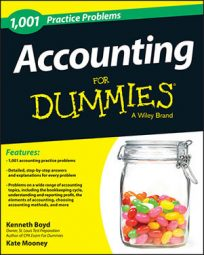##### AccountingAfter you create financial statements, you need some tools to analyze the company’s results. Following are some additional formulas accountants use to analyze financial statements. Become familiar with these formulas, and use them as you practice various accounting problems.

## Burden rate

Fixed manufacturing costs ÷ units produced

Fixed costs can’t be directly traced to a unit produced. For example, a \$50,000 monthly factory lease must be paid, regardless of the number of units produced in a given month. To assign fixed cost to each unit of product, companies used the burden rate.

## Cost of idle capacity

Percentage of available capacity unused × fixed manufacturing overhead costs

Idle capacity refers to unused capacity. Assume you could produce 20% more baseball gloves this month, using your existing factory costs (materials, labor, and overhead). Say that the factory pays a foreman \$50,000 in salary and benefits to supervise production. The cost of idle capacity is 20% multiplied by \$50,000, or \$10,000. The firm is paying an extra \$10,000 for production capacity it’s not using.

## Calculating loan interest

Interest rate for period × principal amount of loan

Interest can be compounded (computed) annually, monthly, or even daily. Pay attention to the stated annual interest rate on the loan and how often interest is compounded. If a 12% loan is compounded monthly, the monthly interest rate is 12% ÷ 12 months, or 1%.

## Effective interest rate

Interest paid ÷ principal amount owed

Because of the effects of compounding, the actual interest paid on a loan may be different from the stated interest rate on the note multiplied by the principal.

## Present value and future value factors

Present value factor less than 1; future value factor more than 1

A present value factor discounts a cash flow to its present value. To calculate the present value, you multiply the factor times the cash flow amount. A present value factor will be less than 1. The future value of a cash flow adjusts the cash flow to its future value, given an interest rate. A future value factor will always be more than 1.

## Return on investment (ROI)

Profit (net income) from investment ÷ cost of investment

A more complex version of the formula is operating income divided by operating assets. Operating assets represent an investment in a project or business. The purpose of this formula is to determine the profitability of a given project.

## Return on investment (DuPont model)

Profit margin × asset turnover

This is a more complex formula that’s used for ROI. Profit margin is operating profit divided by sales. Asset turnover is calculated as sales divided by average assets. Average assets refers to assets at beginning of period + assets balance at end of period ÷ 2.

## Return of investment to shareholders

Retained earnings balance – payments to shareholders

A dividend is a payment of retained earnings to shareholders (investors). If a company makes payments to shareholders that are greater than the balance of retained earnings, those payments are a return of the investors’ original investment.

## Rule of 72

72 ÷ rate of return on investment

The rule of 72 states how many years it will take for a sum of money to double, given a rate of return that is compounded each year. If, for example, the rate of return is 8%, a sum of money will double in 72÷8, or 9 years.

## Weighted average cost of capital (WACC)

Annual cost to obtain financing ÷ capital balance

Companies can raise funds by issuing debt or equity. Outstanding debt requires annual interest payments. Shareholders who purchase equity may also insist on required annual dividend payments. Interest payments on debt and dividend payments to shareholders are both considered financing costs. The annual cost of financing divided by the funds raised to operate the business (capital) is WACC.